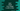# Dart program to check if an integer is odd or even## Introduction:

The `int` class in `Dart` contains two properties to check if a number is even or odd. We can use these properties to find out quickly if a number is odd or even. In this tutorial, I will show you how to do that with an example:

### Method 1: By using the `isEven` and `isOdd` properties:

A number is called an even number if it is perfectly divisible by 2. Else, it is called an odd number. The `isEven` and `isOdd` are two boolean properties defined in the `int` class. These properties of an integer can be used to check if a number is even or odd. The value of `isEven` is `true` for an even number. Else, it will be `false`. Similarly, the value of `isOdd` is `true` if it is an odd number, and it will be `false` otherwise.

#### Example program:

In the below example, we are taking one number as input from the user and it prints if the number is odd or even. It uses the `isEven` and `isOdd` properties to check if the number is even or odd.

``````import 'dart:io';

void main() {
int? number;

print("Enter a number : ");

number = int.tryParse(data ?? '-1');

if (number == null) {
print("Invalid input.");
} else if (number.isEven) {
print("\$number is an even number");
} else if (number.isOdd) {
print("\$number is an odd number");
}
}``````

#### Sample Output:

If you run the above program, it will give outputs as below:

``````Enter a number :
12
12 is an even number

Enter a number :
13
13 is an odd number``````

Note that it will also work for negative numbers. For example, -12 will be considered an even number.

### Method 2: By using the modulo operator:

Another traditional way is to use the modulo operator, `%` to check if a number is even or odd. The modulo operator returns the remainder of a division operation. For an even number `n`, the value `n%2` is 0. Similarly, for an odd number `n`, the value of `n%2` is 1.

The following program shows how to use the modulo operator to check if a number is even or odd:

``````import 'dart:io';

void main() {
int? number;

print("Enter a number : ");

number = int.tryParse(data ?? '-1');

if (number == null) {
print("Invalid input.");
} else if (number % 2 == 0) {
print("\$number is an even number");
} else if (number % 2 == 1) {
print("\$number is an odd number");
}
}``````